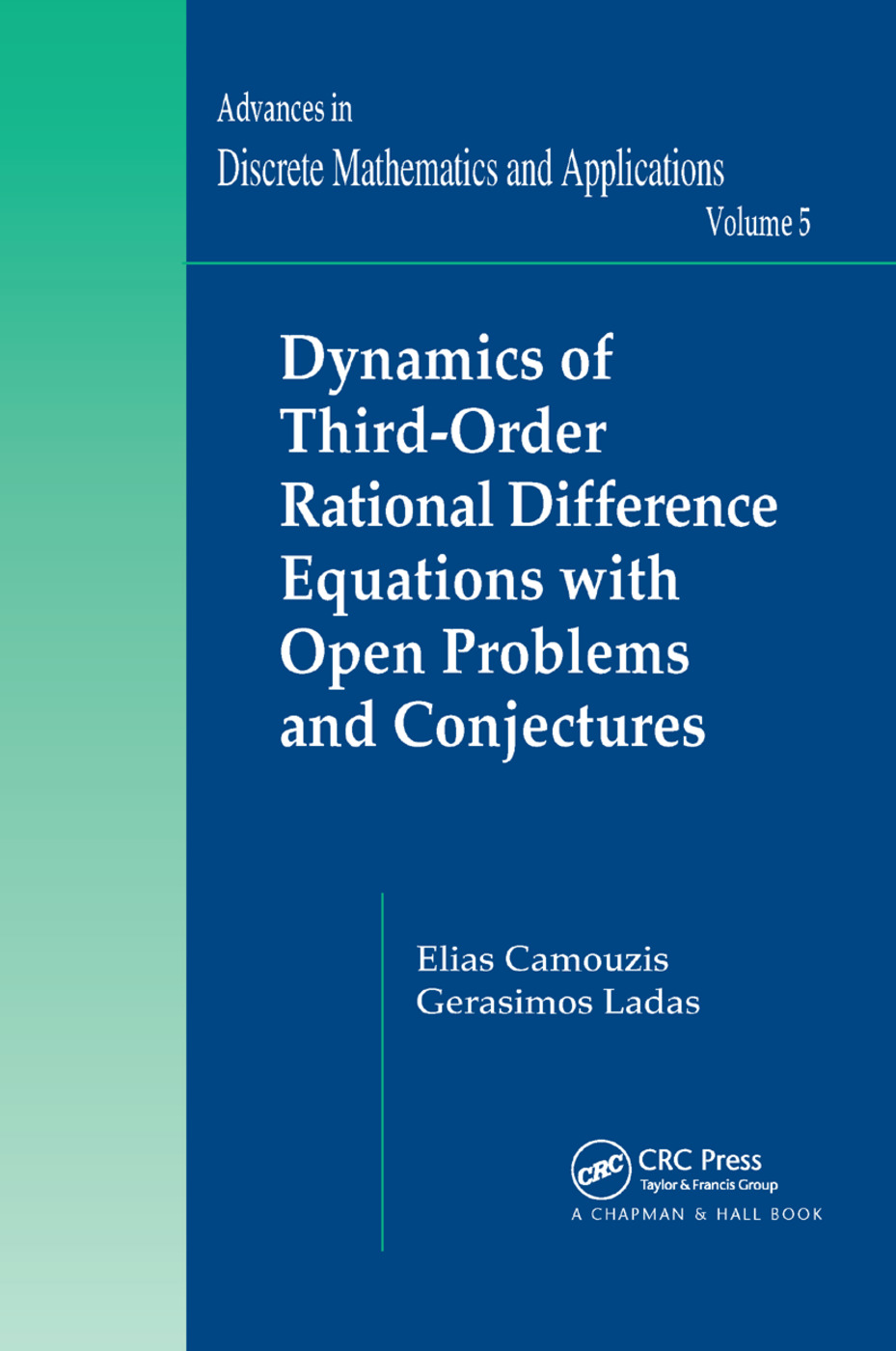# Dynamics of Third-Order Rational Difference Equations with Open Problems and Conjectures

## 1st Edition

Chapman and Hall/CRC

576 pages

Paperback: 9780367388294
pub: 2019-09-05
SAVE ~\$14.99
\$74.95
\$59.96
x
Hardback: 9781584887652
pub: 2007-11-16
SAVE ~\$34.00
\$170.00
\$136.00
x
eBook (VitalSource) : 9780429150302
pub: 2007-11-16
from \$28.98

FREE Standard Shipping!

### Description

Extending and generalizing the results of rational equations,

Dynamics of Third Order Rational Difference Equations with Open Problems and Conjectures focuses on the boundedness nature of solutions, the global stability of equilibrium points, the periodic character of solutions, and the convergence to periodic solutions, including their periodic trichotomies. The book also provides numerous thought-provoking open problems and conjectures on the boundedness character, global stability, and periodic behavior of solutions of rational difference equations.

After introducing several basic definitions and general results, the authors examine 135 special cases of rational difference equations that have only bounded solutions and the equations that have unbounded solutions in some range of their parameters. They then explore the seven known nonlinear periodic trichotomies of third order rational difference equations. The main part of the book presents the known results of each of the 225 special cases of third order rational difference equations. In addition, the appendices supply tables that feature important information on these cases as well as on the boundedness character of all fourth order rational difference equations.

A Framework for Future Research

The theory and techniques developed in this book to understand the dynamics of rational difference equations will be useful in analyzing the equations in any mathematical model that involves difference equations. Moreover, the stimulating conjectures will promote future investigations in this fascinating, yet surprisingly little known area of research.

Preface. Introduction. Preliminaries. Equations with Bounded Solutions. Existence of Unbounded Solutions. Period Trichotomies. Known Results for Each of the 225 Special Cases. Appendices. Bibliography. Index.